# Double Clothesline – Solving EquationsI have always taught solving 2-step linear equations by starting with a balance scale. Having students whittle their way down to see how many marbles were in each bag was always a win for me…..in most cases.I valued this approach. It’s easy to visualize and it strengthens the “whatever you do to one side of an equation you do to the other” mantra we tell students when solving . However, I’ve always been left wanting more especially when we introduce solving equations with negative coefficients or even when the solution is a negative value. The balance scale kinda loses it’s effectiveness.

Using algebra tiles help fill this hole. And now…. thanks to Andrew Stadel, double clotheslines.

I was lucky enough to attend Andrew’s NCTM Annual session on Error Analysis this year. In his session he demonstrated how to use a double clothesline to solve equations. I later found this resource on his site. Watch his videos on how to use the clotheslines….they helped me piece this lesson together. Stop now and go and watch Andrew’s video on solving two step equations.

I stared as Andrew did at the NCTM session:

I put 0 on the top line and 0x on the bottom line.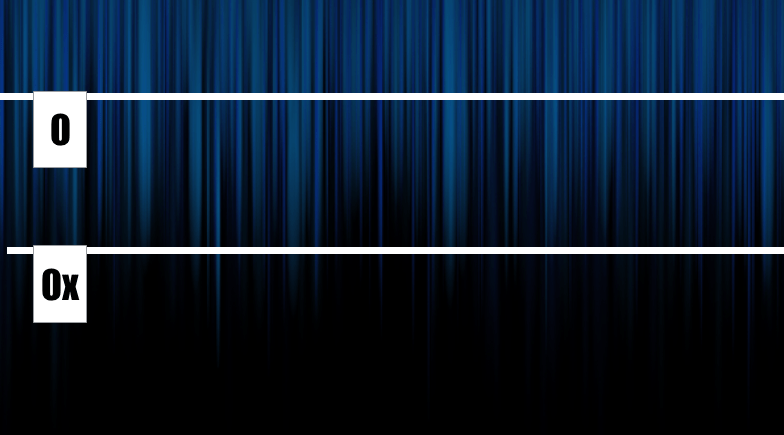I then held up the 3x card and asked where should this go? I asked if it should go on the left or the right of zero. The students overwhelming said it needed to go on the right. “3x is more than x, so it should go more to the right, just like a number line” (Always — Sometimes — Never was going through my head at this moment but i’ll wait to talk about this with the kids until a bit later in the lesson).I then said “I’m going to place this 15 right above the 3x and that means equivalence. 3x is the same as 15”

Where should 9x go? You could see the some students spacing out where 9x should go. This is what I love about this method. It’s so visual and we’re forced to always think about how terms relate to each other.

I want to know what number should be above 9x. I had them draw the number lines on their desks and let them work on determining the value of 9x.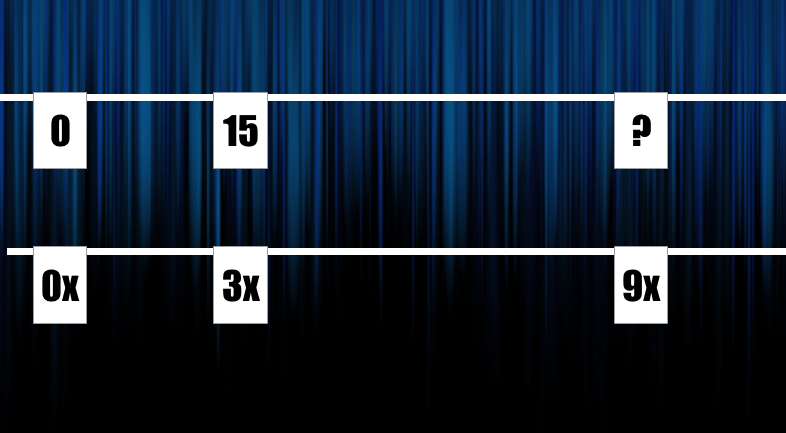Going around the room there were a few different types of solutions. Some students said, “3 times 3x is 9x, so 3 times 15 is 45”

Some students said, “If 3x is 15 one x is 5, so 9x is 45.” Nice. We ensured the whole class understood both of these types.

Next puzzle: I asked where to place 3x + 4…then assigned it the value of 16.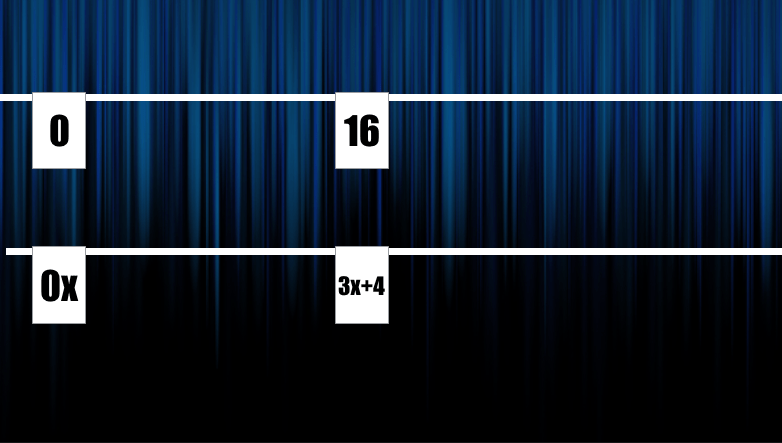Where should 3x be placed? It was easy to see that 3x is less than 3x + 4 so it should go to the left. Now for the amazing moment! What should be the number above?from the class an overwhelmingly 12 was shouted. So now what must be the value of x?Student: “The dividing is the easy part” We spent a few minutes here talking about why dividing 12 by 3 here makes sense.

Next Puzzle:Where should the 5x go? At first some students had some difficulty deciding if it should go to the left or right of 5x – 2.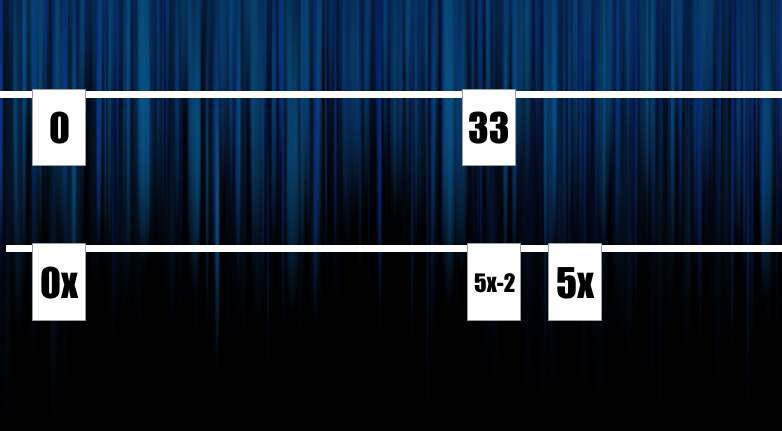Once we settled to the right. They jumped to finishing it off to determine x.

Next Puzzle:Where 3x should go was a discussion. We all agreed it should be 14 down…..and where would that be? This is where the clothesline (number line) feels superior and the balance scale visual falls short. We can use the bi direction of the number line to continue working with negative values.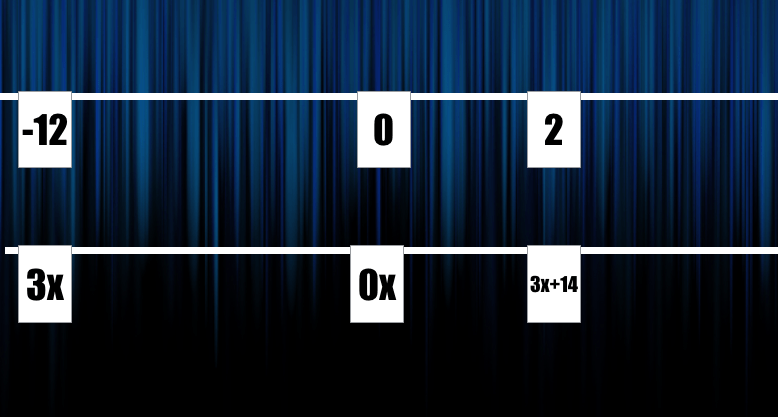What was awesome during this class was this wasn’t a big deal….the number lines seems natural!!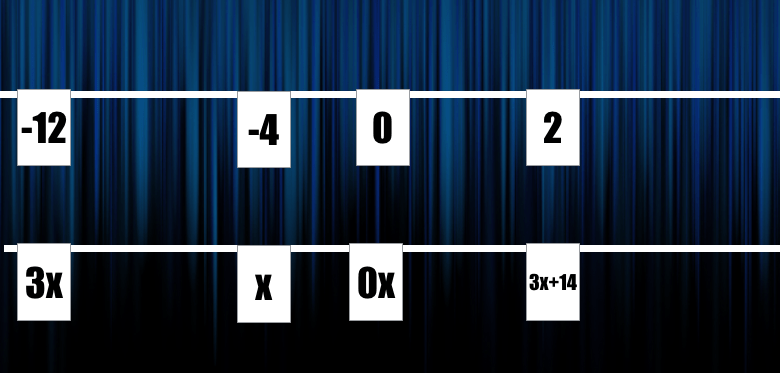Also watch Andrew’s example with negative coefficients.

I had students practice solving a variety of equations by drawing the cards on their handout.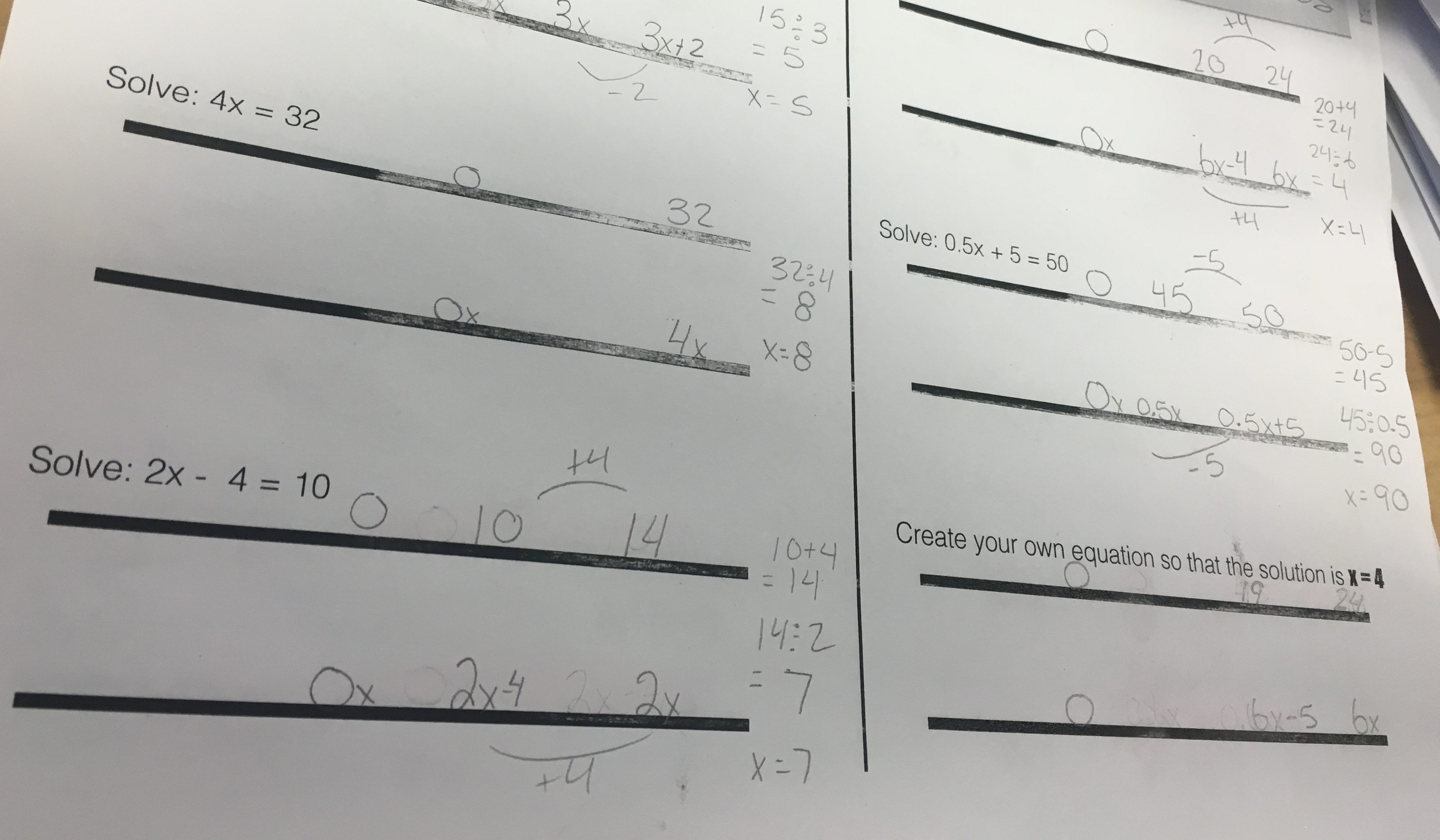They finally demonstrated their understanding by creating their own equation where x had to equal 4. They put their creations up around the room for the group to solve.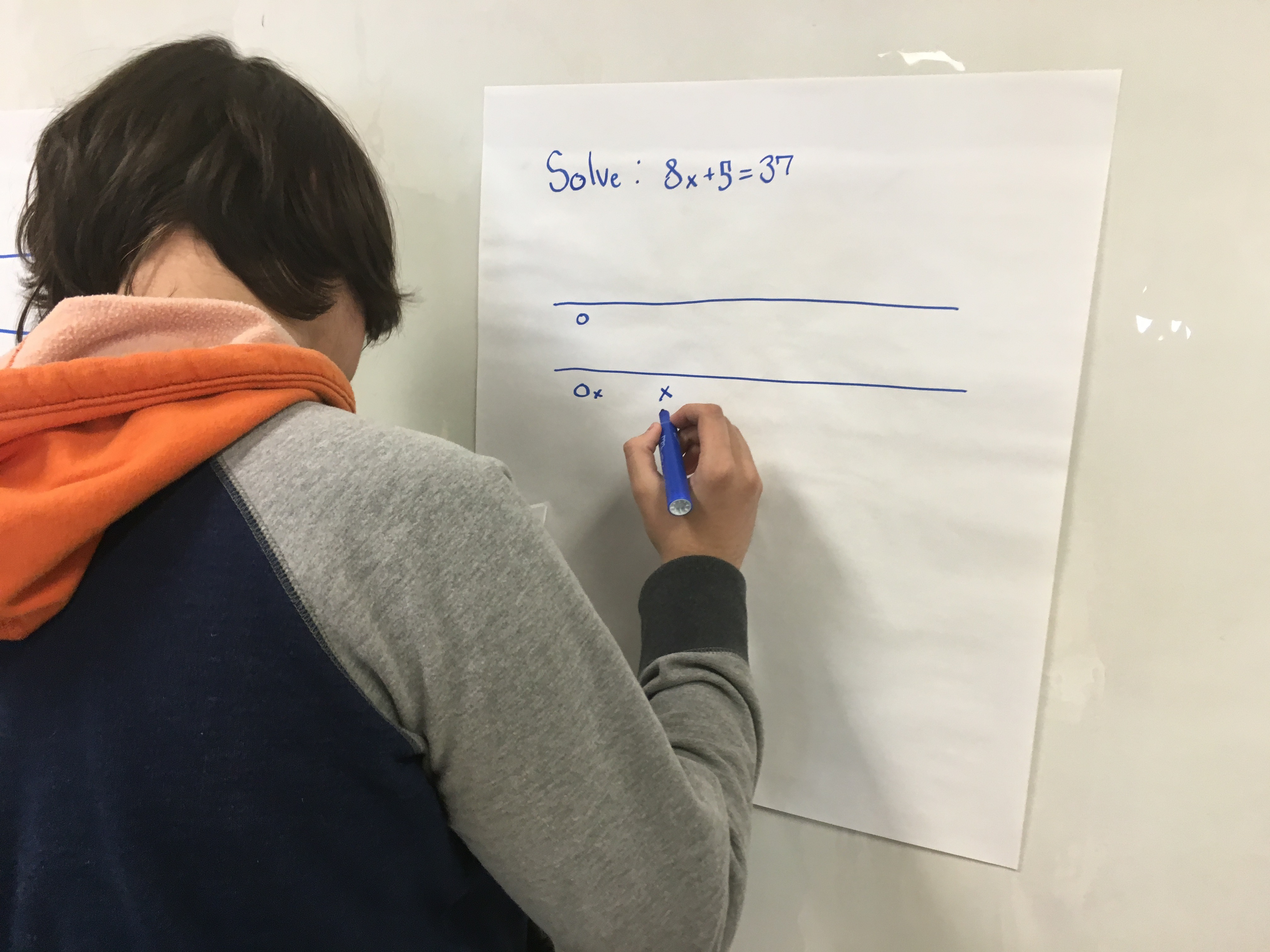I feel that the number line (clothesline) method builds a lot of great number sense. We get to reinforce our inverse operations as we build from conceptual understanding to abstract. Students’ strengthen their understanding of algebraic expressions and how those expressions relate to others.

I’m now going to investigate how to to demonstrate solving multi-step equations…. 3x + 5 = 2x + 7 using the clothesline. I’m thinking this might be a difficult task. Any ideas????

[UPDATE] – Solving equations with expression on both sides.

Since this lesson my class used the double number line to solve equations like 4x + 10 = 6x + 2. It was great to keep some continuity here while we solved harder equations.

We placed each side of the equation on separate clotheslines just like before.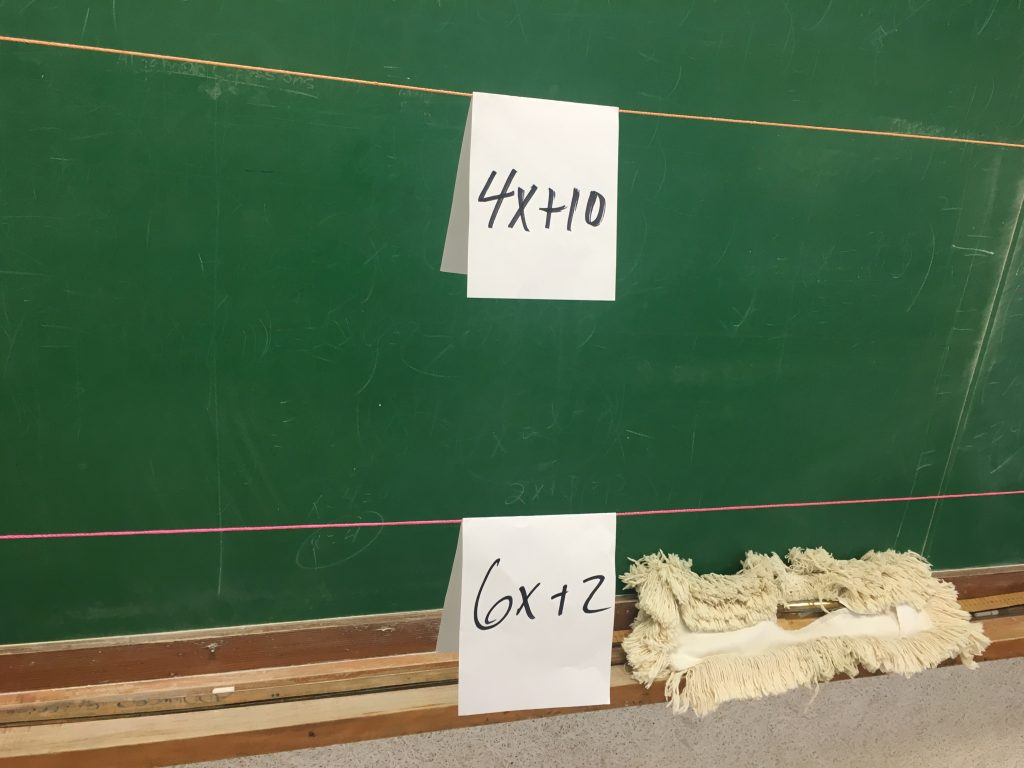We didn’t want to re-invent a new strategy….we were great at solving equations when one line was used for numbers and the other for expressions…..so we wanted that. How can we get one line to be just numbers and one to have the expression? We subtracted 4x from both lines.  Which left us exactly where we were last class!!and then we subtracted 2 from both to isolate the “x-term”Finally dividing by 2Boom!

This will be our method too to solve a system of equations that are both in terms of y.

More clothesline:

## 17 thoughts on “Double Clothesline – Solving Equations”

1.3x+5 on the top number line; 2x+7 on the bottom.

Where does 2x+5 go? Left What would be on bottom? (x+7)
x+5? Left Bottom? 7
x? Left 5 units Bottom?

2.Oh, and I love this. I have been reading Pam Harris’s Building Powerful Numeracy. They will work great in tandem. Her modeling of equations on the number line is a perfect followup to this and this is a natural way to do problem strings for solving equations! Sharing with my PLC…

3.I like Ben’s idea. The first time through, I might stay with the top number line, talking about where 2x + 5 and x + 5 would go, how far apart they are, and how they relate to 5, making sure they really understand how the distance x relates to each gap. (Like Jon did, I would probably revisit negative values in another example. But it would be cool if someone brought it up – you would know they were getting the idea.) And then go down to the second number line and ask what goes under each one.

4.Matt Vaudrey

I’m back in the classroom two periods a day this year. This is happening the first week of school.

1.Jon Orr

Awesome to hear Matt! I’ll be Doing this lesson again for sure!

5.Sandra Oestreicher

Similar to Ben’s above

3x + 5 on the top, 2x + 7 on the bottom. (Don’t think top/bottom choice really matters though)

Similar to solving single step, think about making one of the expressions with the variable term only

I ‘adjusted’ the top by moving it to the left 5 units (symbolically) leaving 3x so to keep the equivalence the expression on the the bottom must also move 5 units to the left making it 2x + 2

Now ‘adjust’ the x-terms….move left by subtracting 2x from both expressions and the result is x on the top line being equivalent to 2 on the bottom line

1.Jon Orr

Thanks Sandra! Last semester I played around. In my class we manipulated one “line” to eliminate the variable part…which left us just like the examples above. For example we would have subtracted 2x from both “lines” leaving x+5 = 7….lots of different ways!

6.Angela Schaefer

I am going to try this for the first time this week. I like the concept and I am interested in how it will develop my students’ understanding. Right at the top of this post you had a link to Andrew’s video on two-step equations. I used this myself last week to understand and it was excellent. I clicked on it today to show one of my colleagues and I am sure this is not the same video… it doesn’t demonstrate equations. Do you know where the other video might be so I could show it? Thanks for responding.

Ps– Like the ideas for multi-step equations…

1.Jon Orr

Hi Angela, Andrew has taken the link down temporarily. I’ll let you know when it’s back up.

1.Tim Pitkin

We spent two days on this with an SEI Alg. 1 class comprised of students with extremely diverse educational backgrounds and ages. We have refugees who had one year of formal schooling before arriving with us to others who have had years of formal education. Add to the mix 15 to 21 year olds, in yes a public HS.

Anyway, we found that the two clotheslines provided for an almost infinite number of entry points into the conversation. Because it such a large class, 22 students, we used a lot of think-pair-share. It was absolutely astonishing to have so many students enthusiastically solving equations!
We discovered that maintaining proportionality or distance was critical for discussion. We’re also finding this to be a powerful strategy for discussion structure and what does it mean to add or subtract a value. I think many, including myself, have never solved linear equations on a line before.
One problem for tomorrow is 3x + 4 = 16. The second card and first question is where does 2x + 14 belong and why. The next is to have a ? above 2x + 14 and ask if they can determine the value here and why or why not.
Another problem is 3x + 12 = 2. After working with the negative, the question might be what must 3x + 12 have to equal in order for the solution to be positive?

7.Tim Pitkin

Just came upon your blog and am trying this tomorrow in an SEI Alg. 1. I’m going to start with variables on one side as review and to build the concept of the clothesline and then move into variables on both sides. I’ll let you know how it works.

8.I used these lessons with my 7th graders after we used bags and coins. I am amazed at how quickly every single student picked up the method…and was pretty quickly able to transition to writing the steps to solve. I am a believer! Next up, I want to use this sam method to add in solving when the distributive property is involved. I am debating either 3 lines, (the new one for the step to address he distribute property) or just putting that step on top of the card one we manipulate the Distilled Prop. If anyone has a better idea, I would LOVE to hear it! I have used Algeblocks in the past, but my kids took to this…I would love to see it though!

9.Tim Pitkin

Since my 9th graders had already studied the distributive property, I kept the two lines. I wanted to maintain the structure of equality so we did this: 5(x + 2) = 3x + 15. The next card I showed was 5x + 10 and asked where it belonged. There was great discussion about this. Some said to the right, others to the left before someone saw they were equal and that it belonged atop 5(x + 2).

1.Thank you, and sorry for the many typos. I will follow your advice! We have worked on the distributive property previously, but never within an equation. I think your idea gets at this. We are also studying equality as well, equivalent expressions and this will accomplish more than just one goal. Thank you again!

10.Jon Orr

Thanks for the suggestion Tim. That’s what I’ve done in the past too. Showing equality is powerful. I don’t think it needs another clothesline for it.

11.Sonya Terry

Have you tried using this idea to teaching inequalities? If so, can you give me pointers. I’m really loving the concept but I’m having a difficult time with teaching it for inequalities and dividing by that crazy negative sign. Any help would be appreciated.

12.This is awesome! Do you have handouts that you’d be willing to share? I see you using some with the students…